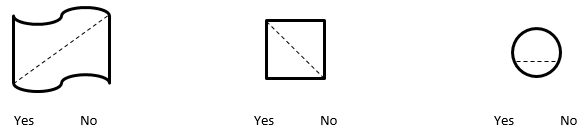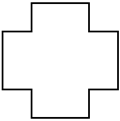# Shapes and Angles

## Objective

Identify and draw lines of symmetry in two-dimensional figures.

## Common Core Standards

### Core Standards

?

• 4.G.A.3 — Recognize a line of symmetry for a two-dimensional figure as a line across the figure such that the figure can be folded along the line into matching parts. Identify line-symmetric figures and draw lines of symmetry.

## Criteria for Success

?

1. Understand that a line of symmetry is a line through a figure such that when the figure is folded along the line, two halves are created that match up exactly.
2. Understand that a symmetric shape is a shape for which at least one line of symmetry exists.
3. Identify whether a given line is a line of symmetry or not.
4. Draw lines of symmetry in a figure.
5. Understand the special case that circles have an infinite number of lines of symmetry, all of which go through its center.
6. Given half of a symmetric shape and its line of symmetry on grid paper, draw the whole symmetric shape (MP.6).

## Tips for Teachers

?

• The following material is needed for today's lesson: Scissors

#### Remote Learning Guidance

If you need to adapt or shorten this lesson for remote learning, we suggest prioritizing Anchor Task 2 (benefits from worked example). Find more guidance on adapting our math curriculum for remote learning here.

#### Fishtank Plus

• Problem Set
• Student Handout Editor
• Vocabulary Package

?

### Problem 1

All of the following cutout shapes were made the same way, by folding a piece of paper before cutting. Where do you think the paper was folded to make each of the following shapes? Draw a line to show your thinking.### Problem 2

Find the line(s) of symmetry in the following geometric figures.

a.b.c.d.e.### Problem 3

Garret drew a rhombus on grid paper, and then folded the paper along the rhombus’ line of symmetry. Draw what the grid paper looks like folded.Felicia drew part of a shape on grid paper. The folded edge is the full shape’s line of symmetry. Draw what the grid paper looks like unfolded.## Problem Set & Homework

#### Discussion of Problem Set

• In #2, which figures had lines of symmetry that were most difficult to see? Why were some easier and others more difficult?
• In #3, why does a circle have an infinite number of lines of symmetry?
• In #4, how many lines of symmetry did the equilateral triangle have? The isosceles triangle? The scalene triangle? Do you think that is true of all triangles of those types? Why or why not?
• In #5, what method did you use to complete each figure? How would you complete the figure if there were no graph paper?
• Identify objects around the classroom or in nature that have lines of symmetry.

?

### Problem 1

Is the line drawn a line of symmetry? Circle your choice.#### References

EngageNY Mathematics Grade 4 Mathematics > Module 4 > Topic D > Lesson 12Exit Ticket, Question #1

Grade 4 Mathematics > Module 4 > Topic D > Lesson 12 of the New York State Common Core Mathematics Curriculum from EngageNY and Great Minds. © 2015 Great Minds. Licensed by EngageNY of the New York State Education Department under the CC BY-NC-SA 3.0 US license. Accessed Dec. 2, 2016, 5:15 p.m..

### Problem 2

Draw as many lines of symmetry as you can find in the figure below.#### References

EngageNY Mathematics Grade 4 Mathematics > Module 4 > Topic D > Lesson 12Exit Ticket, Question #2

Grade 4 Mathematics > Module 4 > Topic D > Lesson 12 of the New York State Common Core Mathematics Curriculum from EngageNY and Great Minds. © 2015 Great Minds. Licensed by EngageNY of the New York State Education Department under the CC BY-NC-SA 3.0 US license. Accessed Dec. 2, 2016, 5:15 p.m..

### Mastery Response

?